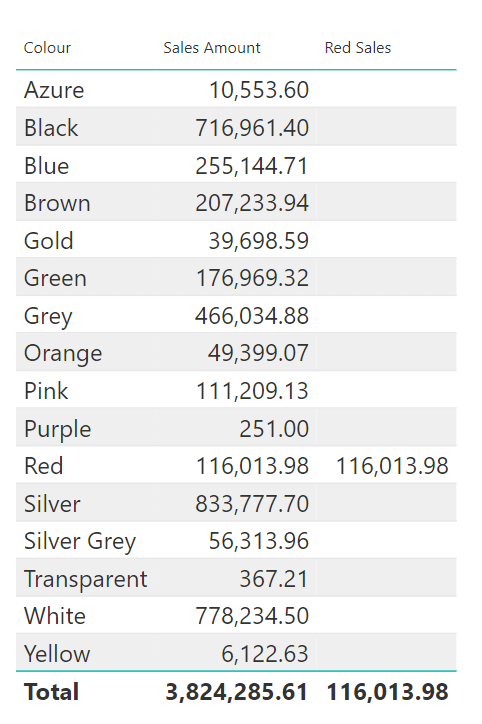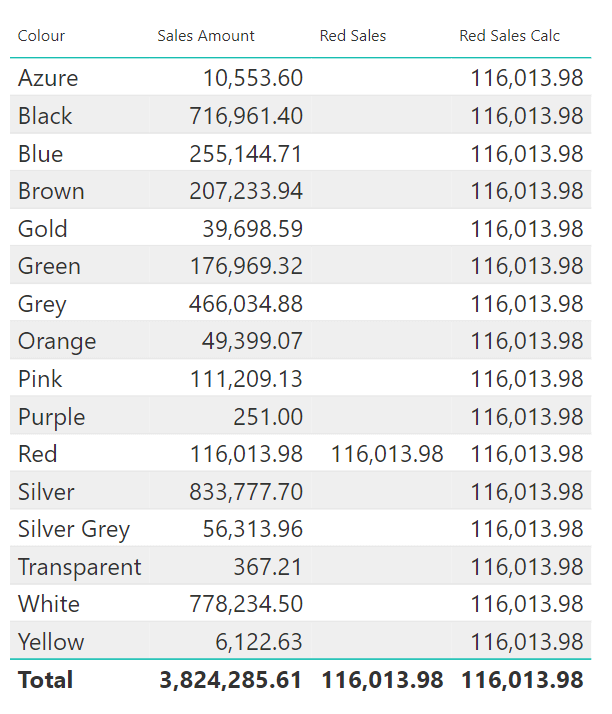## What is CALCULATE?

`CALCULATE` (and `CALCULATETABLE`) is the only function in DAX that has the power of changing and updating the filter context.

The filter context active in each cell of a report is generated by:

• Row Selection.
• Column Selection.
• Slicer Selection.
• Report Filters.

`CALCULATE` can be used to alter the filter context coming from the report through columns, rows, slicers etc. Inside `CALCULATE`, you can change and update this filter context and then compute values on top of it.

## How does CALCULATE work?

The syntax of `CALCULATE` is very simple: it is just `CALCULATE`, followed by an expression and then a set of filters. The filters are evaluated, and their result can change the filter context. Finally, once all the filters have been evaluated and the new filter context has been created, then `CALCULATE` will evaluate the expression in the new filter context.

When we use the following expression in our Power BI report to compute the sales amount of red products:

``````Red Sales =
SUMX (
FILTER (
Sales,
RELATED ( 'Product'[Colour] ) = "Red"
),
Sales[Quantity] * Sales[Net Price]
)
``````

The inner `FILTER` function is returning the 'Sales' table but filtered so that only the rows where the 'Product' colour is 'Red' are returned. `SUMX` then iterates over this filtered table to calculate the `Sales[Quantity] * Sales[Net Price]` for each row in the table.

When applied to the table visual in the report we only see the value for 'Red Sales', in the 'Red' row of the report. This is because the 'Red Sales' measure takes into account the external filter context applied in each row of the report.

In the first row, the filter context is 'Azure', and the second row the filter context is 'Black' and so forth. As we are filtering the dataset using `RELATED ( 'Product'[Colour] ) = "Red"` in our expression, the resulting dataset for these rows is empty and the cell is blank. The value for 'Red Sales' is only seen when 'Red' is already selected in the external filter context.When we use the following expression:

``````Red Sales Calc =
CALCULATE (
SUMX (
Sales,
Sales[Quantity] * Sales[Net Price]
),
'Product'[Colour] = "Red"
)
``````

When this measure is applied to the table visual in the report, we can see that `CALCULATE` always computes the same value for each row. This is because in every row, the external filter context of 'Colour' has been replaced by `'Product'[Colour] = "Red"` supplied to `CALCULATE`. So the value returned is always the sales amount of red products.`CALCULATE` places a filter on the whole model. When you put a filter on a table, this filter propagates through to other tables and is applied to the whole model. So the filter `'Product'[Colour] = "Red"` is applied on the 'Colour' column of the 'Product' table and this propagates automatically. Then we are iterating and computing values with `SUMX` on the 'Sales' table.

## Conclusion

So, to summarise `CALCULATE` computes an expression in a new filter context which is modified by what you provide as a filter. It is the only function in the whole DAX language that has the power of changing the filter context.

### Jessica Hill

#### Apprentice Engineer IIIJessica comes from a Biosciences background, having gained a 1st Class Bachelor of Science in Biology from The University of Manchester.

During the lockdown Jessica used the opportunity to explore her interest in technology by taking two Code First Girls online courses; Introduction to Web Development and Python Programming.

This led Jessica to look for job opportunities in the technology sector and joined endjin's 2021 apprenticeship cohort, which had over 200 applicants.